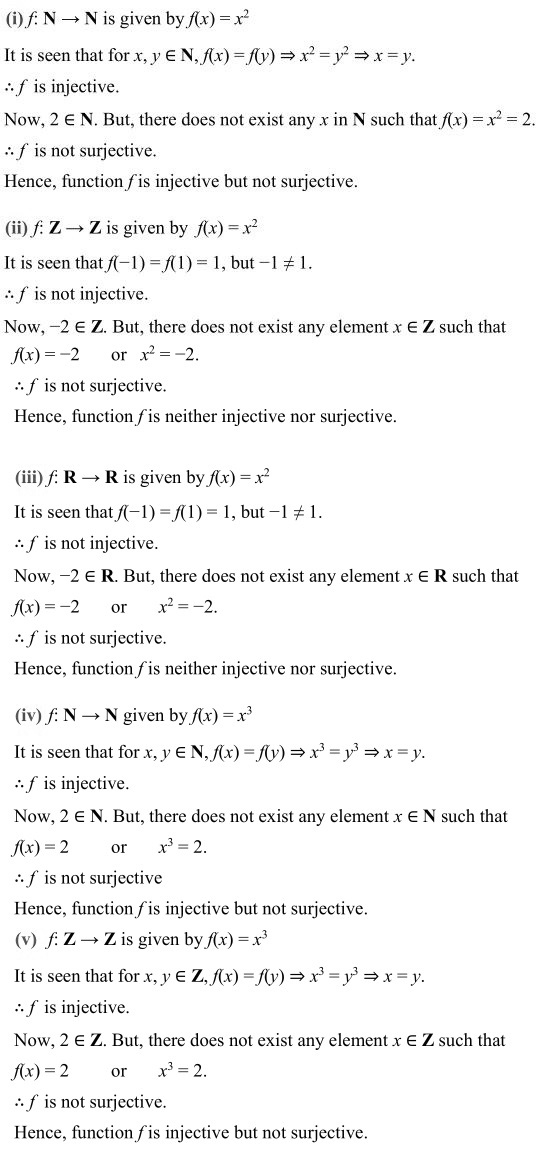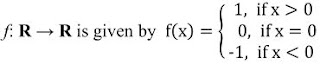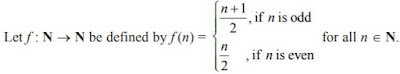#### NCERT Solutions for Class 12th: Ch 1 Relations and Functions Exercise 1.2 Math

Page No: 10

Exercise 1.2

1. Show that the function f : R∗ → R∗ defined by f(x) = 1/x is one-one and onto, where R∗ is the set of all non-zero real numbers. Is the result true, if the domain R∗ is replaced by N with co-domain being same as R∗?2. Check the injectivity and surjectivity of the following functions:
(i) f : N → N given by f(x) = x2
(ii) f : Z → Z given by f(x) = x2
(iii) f : R → R given by f(x) = x2
(iv) f : N → N given by f(x) = x3
(v) f : Z → Z given by f(x) = x33. Prove that the Greatest Integer Function : R → R, given by f(x) = [x], is neither one-one nor onto, where [x] denotes the greatest integer less than or equal to x.

: R → R is given by, f(x) = [x]
It is seen that f(1.2) = [1.2] = 1
f(1.9)= [1.9] = 1
f(1.2) = f(1.9), but 1.2 ≠ 1.9
f is not one-one.
Now, consider 0.7 ∈ R.
It is known that f(x) = [x] is always an integer.
Thus, there does not exist any element x ∈ R such that f(x) = 0.7.
f is not onto.
Hence, the greatest integer function is neither one-one nor onto.

Page No. 11

4. Show that the Modulus Function f : R → R, given by f(x) = |x|, is neither one-ne nor onto, where |x| is x, if x is positive or 0 and |x| is – x, if x is negative.It is clear that f(-1) = I-1| = 1
and f(1) = I1l = 1
f(-1) = f(1), but -1 ≠ 1
f is not one-one.
Now, consider -1 ∈ R.
It is known that f(x) = Ix| is always non-negative.
Thus, there does not exist any element x in domain R such that f(x) = Ix| = -1.
∴ f is not onto.
Hence, the modulus function is neither one-one nor onto.

5. Show that the Signum Function f : R → R, given byIt is seen that f(1) = f(2) = 1, but 1  ≠ 2.
∴ f is not one-one.
Now, as f(x) takes only 3 values (1, 0, or -1) for the element -2 in co-domain R, there does not exist any x in domain R such that f(x) = -2.
f is not onto.
Hence, the Signum function is neither one-one nor onto.

6. Let A = {1, 2, 3}, B = {4, 5, 6, 7} and let f = {(1, 4), (2, 5), (3, 6)} be a function from A to B. Show that f is one-one.

It is given that A= (1, 2, 3), B= (4, 5, 6, 7).
A → B is defined as = {(1, 4), (2, 5), (3, 6)}
f(1)= 4,
f(2) = 5,
f(3) = 6
It is seen that the images of distinct elements of A under f are distinct. Hence, function f is one-one.

7. In each of the following cases, state whether the function is one-one, onto or bijective. Justify your answer.
(i) f : R → R defined by f(x) = 3 - 4x
(ii) f : R → R defined by f(x) = 1+x2

(i) f : R → 12 is defined as f(x) = 3 - 4x.
Let x1, x2 ∈ R such that f(x1) = f(x2
⇒ 3-4x1 = 3-4x2
⇒ -4x1 = -4x
⇒ x1 = x2
∴ f is one-one.∴ f is onto.
Hence, f is bijective.

(ii) f: R → R is defined as f(x) = 1 + x2
Let x1, x2 ∈ R such that f(x1) = f(x2)
⇒ 1+ x12 = 1 + x22
⇒ x12 = x22
⇒ x1 = ± x2
∴ f(x1) = f(x2) does not imply that x1 = x2

8. Let A and B be sets. Show that f : A×B → B×A such that f(a, b) = (b, a) is bijective function.

f: A × B → B × A is defined as f(a, b) = (b, a).
Let (a1, b1), (a2, b2) ∈ A × B such that f(a1, b1) = f(a2, b2)
⇒ (b1, a1) = (b2, a2)
⇒ b1 = b2 and a1 = a2
⇒ (a1, b1) = (a2, b2)
∴ f is one – one.
Now, let (b, a) ∈ B × A be any element.
Then, there exists (a, b) ∈ A × B such that f(a, b) = (b, a). [By definition of f]
∴ f is onto.
Hence, f is bijective.

9.10. Let A = R – {3} and B = R – {1}. Consider the function f : A → B defined by f(x) = (x-2)/(x-3).  Is f one-one and onto? Justify your answer.11. Let f : R → R be defined as f(x) = x4. Choose the correct answer.
(A) f is one-one onto
(B) f is many-one onto
(C) f is one-one but not onto
(D) f is neither one-one nor onto.

f: R → R is defined as f(x) = x4
Let x, y ∈ R such that f(x) = f(y).
⇒ x4 = y4
⇒ x = ± y
∴ f(x) = f(y) does not imply that x = y.
For example f(1) = f(–1) = 1
∴ f is not one-one.
Consider an element 2 in co-domain R. It is clear that there does not exist any x in domain R such that f(x) = 2.
∴ f is not onto.
Hence, function f is neither one – one nor onto.

12. Let f : R → R be defined as f(x) = 3x. Choose the correct answer.
(A) f is one-one onto
(B) f is many-one onto
(C) f is one-one but not onto
(D) f is neither one-one nor onto.

f: R → R is defined as f(x) = 3x.
Let x, y ∈ R such that f(x) = f(y).
⇒ 3x = 3y
⇒ x = y
∴f is one-one.
Also, for any real number (y) in co-domain R, there exists y/3 in R such that f(y/3) = 3(y/3) = y
∴ f is onto.
Hence, function f is one – one and onto.